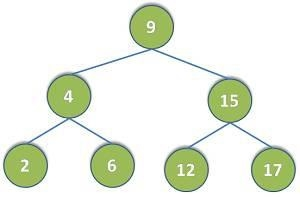# Write a program to Delete a Tree in C programming

To delete a tree we need to traverse each node of the tree and then delete each of them. this one by one delete every node of the tree and makes it empty. For this, we need to use a method that traverses the tree from bottom to up so that we can delete the lower notes first and then their parents so that no extra complexities arise. Based on the condition that we need, The postorder traversal will be the best suited And works efficiently so that our program will be optimum.

The post-order for the following tree is -

2-6-4-12-17-15

The postorder travel cell technique works in by the following way -

checks for the left childnode → check for the rootnode → chekcs the right childnode## Example

#include<stdio.h>
#include<stdlib.h>
struct node {
int data;
struct node* left;
struct node* right;
};
struct node* node = (struct node*)
malloc(sizeof(struct node));
node->data = data;
node->left = NULL;
node->right = NULL;
return(node);
}
void nodedel(struct node* node) {
if (node == NULL) return;
nodedel(node->left);
nodedel(node->right);
printf(" Node deleted, value is %d", node->data);
free(node);
}
int main() {
nodedel(root);
root = NULL;
printf(" Tree deleted ");
return 0;
}

## Output

Node deleted, value is 4
Node deleted, value is 12
Node deleted, value is 17
Node deleted, value is 15
Node deleted, value is 9
Tree deleted